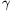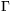Mathematical and Physical Journal
for High Schools
Issued by the MATFUND Foundation
 Already signed up? New to KöMaL?

#Problem A. 468. (December 2008)

A. 468. We are given two triangles. Their side lengths are a,b,c and A,B,C respectively, the areas are t and T, respectively. Prove that -a2A2+a2B2+a2C2+b2A2-b2B2+b2C2+c2A2+c2B2-c2C216tT.

(5 pont)

Deadline expired on January 15, 2009.

Solution. Letandthe two angles opposite to sides c and C, respectively. From the cosine law we have a2+b2-c2=2abcosand A2+B2-C2=2ABsin; the two areas areand.

Substituing these quantities,

-a2A2+a2B2+a2C2+b2A2-b2B2+b2C2+c2A2+c2B2-c2C2-16tT=

=2a2B2+2A2b2-(a2+b2-c2)(A2+B2-C2)-16tT=

=2a2B2+2A2b2-4abABcoscos-4abABsinsin=

=2(aB-Ab)2+4abAB(1-cos (-))0.

### Statistics:

 12 students sent a solution. 5 points: Blázsik Zoltán, Bodor Bertalan, Éles András, Márkus Bence, Nagy 235 János, Nagy 648 Donát, Somogyi Ákos, Tomon István, Tossenberger Anna, Varga 171 László, Wolosz János. 4 points: Backhausz Tibor.

Problems in Mathematics of KöMaL, December 2008